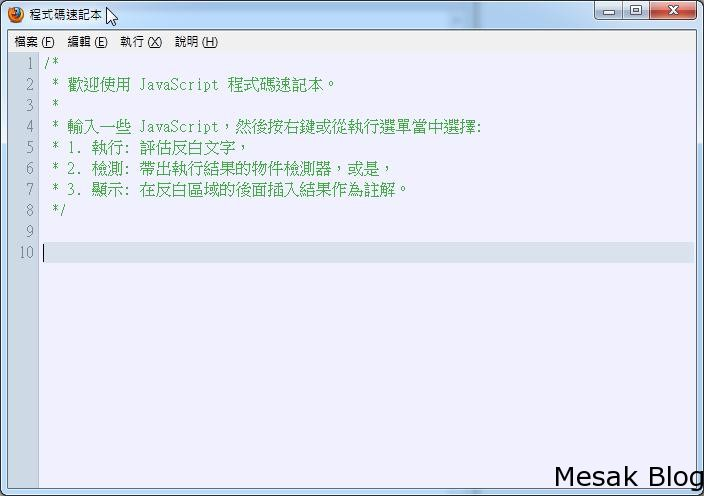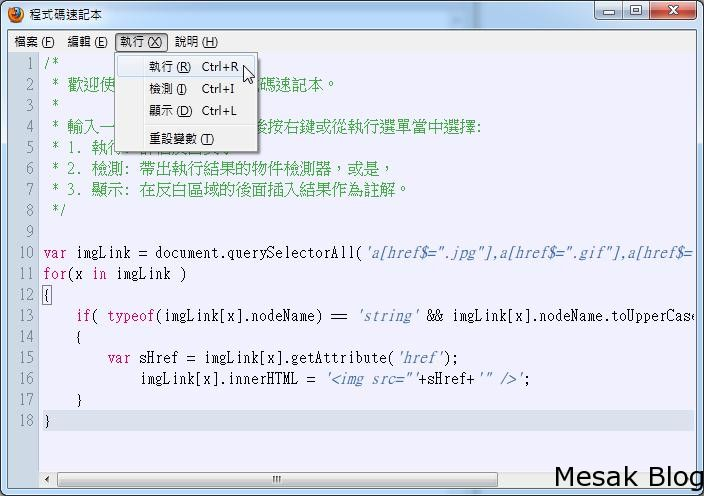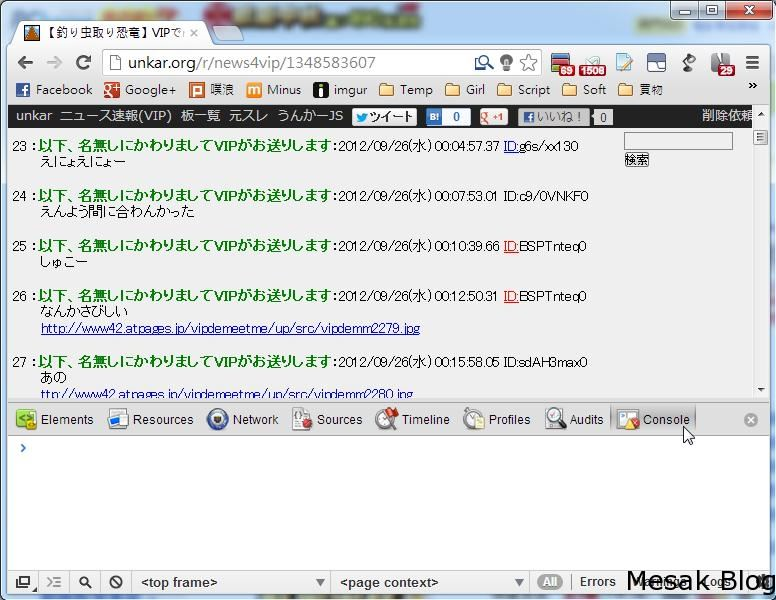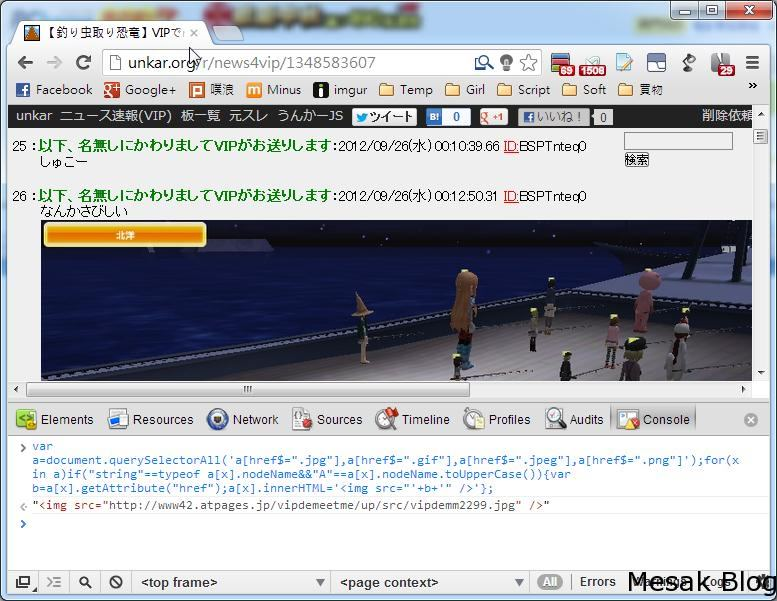# [教學] 如何把文章中的連結變成圖片直接看

firefox 在更新到某一版的時候(我忘了，就新增了新功能 “程式碼速記本”{
{
}
}Chrome 沒有程式碼速記碼，但是有控制台var a=document.querySelectorAll(‘a[href\$=”.jpg”],a[href\$=”.gif”],a[href\$=”.jpeg”],a[href\$=”.png”]’);for(x in a)if(“string”==typeof a[x].nodeName&&”A”==a[x].nodeName.toUpperCase()){var b=a[x].getAttribute(“href”);a[x].innerHTML='<img src=”‘+b+'” />’}javascript:(function(){var a=document.querySelectorAll(‘a[href\$=”.jpg”],a[href\$=”.gif”],a[href\$=”.jpeg”],a[href\$=”.png”]’);for(x in a)if(“string”==typeof a[x].nodeName&&”A”==a[x].nodeName.toUpperCase()){var b=a[x].getAttribute(“href”);a[x].innerHTML='<img src=”‘+b+'” />’};})()